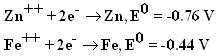# Electrochemistry Quiz: MCQ Exam! Trivia

15 Questions | Total Attempts: 1455SettingsRelated Topics
• 1.
Which of the following decreases with dilution:
• A.

Molar Conductance

• B.

Conductance

• C.

Specific Conductance

• D.

Equivalent Conductance

• 2.
An increase in equivalent conductance of a strong electrolyte with dilution is mainly due to:
• A.

Increase in number of ions

• B.

Increase in ionic mobility of ions

• C.

100% ionisation of electrolyte at normal dilution

• D.

Increase in both i.e., number of ions and ionic mobility of ions

• 3.
• A.

The standard reduction potential of Li+/Li is lower than that of Cu+/Cu

• B.

The standard reduction potential of Cu+/Cu is lower than that of Li+/Li

• C.

The standard oxidation potential of Li+/Li is lower than that of Cu+/Cu

• D.

Li is smaller in size as compared to Cu

• 4.
The standard electrode potential is measured by:
• A.

Electrometer

• B.

Voltmeter

• C.

Pyrometer

• D.

Galvanometer

• 5.
• A.

KNO3 is highly soluble in water

• B.

Velocity of (NO3)- is greater than that of K+

• C.

Velocity of K+ is greater than that of (NO3)-

• D.

Velocity of both K+ and (NO3)- are nearly the same

• 6.
What would happen if the electrodes used in the electrolysis process rective:
• A.

Electrode does not participate in the chemical reaction

• B.

Electrodes only act as source and sink for electrons

• C.

Electrodes participate in the electrode reaction

• D.

Electrodes are burnt up

• 7.
9.65 C of electric current is passed through fused anhydrous magnesium chloride. The magnesium metal thus, obtained is completely converted into a Grignard reagent. The number of moles of the Grignard reagent obtained is:
• A.

5 x 10-4

• B.

1 x 10-4

• C.

5 x 10-5

• D.

1 x 10-5

• 8.
Resistance of a conductive cell filled with a solution of an electrolyte of concentration 0.1 M is 100 Ω. The conductivity of this solution is 1.29 S m–1. Resistance of the same cell when filled with 0.2 M of the same solution is 520 Ω. The molar conductivity of 0.02 M solution of the electrolyte will be:
• A.

1240 x 10-4 S m2 mol-1

• B.

124 x 10-4 S m2 mol-1

• C.

12.4 x 10-4 S m2 mol-1

• D.

1.24 x 10-4 S m2 mol-1

• 9.
The reduction potential of hydrogen half-cell will be negative if:
• A.

P(H2) = 1 atm and [H+] =  M

• B.

P(H2) = 1 atm and [H+] = 2 M

• C.

P(H2) = 2 atm and [H+] = 1 M

• D.

P(H2) = 2 atm and [H+] = 2 M

• 10.
The hydrogen electrode is dipped in a solution of pH 3 at 25°C. The potential would be (the value of 2.303 RT/F is 0.059 V)
• A.

0.177 V

• B.

- 0.177 V

• C.

0.059 V

• D.

- 0.059 V

• 11.
Which of the following reaction is preferred during electrolysis of dilute sulphuric acid:
• A.

2H2O (l) → O2 (g) + 4H+ (aq) + 4e-

• B.

2SO42- (g) → S2O82- (g) + 2e-

• C.

2H2 (g) + 4OH- (aq) → 4H2O (l) + 4e-

• D.

2SO42- (aq) → S2O82- (aq) + 2e-

• 12.
The standard electrode potential for the half cell reactions are: The E.M.F. of the cell reaction
• A.

– 1.20 V

• B.

+ 1.20 V

• C.

+ 0.32 V

• D.

– 0.32 V

• 13.
Al2O3 is reduced by electrolysis at low potentials and high currents. If 4.0 × 104 amperes of current is passed through molten Al2O3 for 6 hours, what mass of aluminum is produced? (Assume 100% current efficiency, atomic mass of Al = 27 g/mol)
• A.

9.0 x 103 g

• B.

8.1 x 104 g

• C.

2.4 x 105 g

• D.

9.0 x 103 g

• 14.
When KMnO4 acts as an oxidizing agent and ultimately forms MnO42–, MnO2, Mn2O3 and Mn2+, then the number of electrons transferred in each case is:
• A.

1, 3, 4, 5

• B.

3, 7, 1, 5

• C.

4, 3, 1, 5

• D.

1, 5, 7, 3

• 15.
For a cell reaction involving two electrons change, the standard E.M.F. of the cell is found to be 0.295 V at 25°C. The equilibrium constant of the reaction at 25°C is:
• A.

2.95 x 10-2

• B.

2.95 x 102

• C.

1 x 1010

• D.

1 x 10-10